# i. The speed of a separately excited dc motor is controlled by a one-phase full-wave converter. The field circuit is also controlled by a full-wave converter, see V supply) Vs pk-150V Ra-0.310 L Rf 1...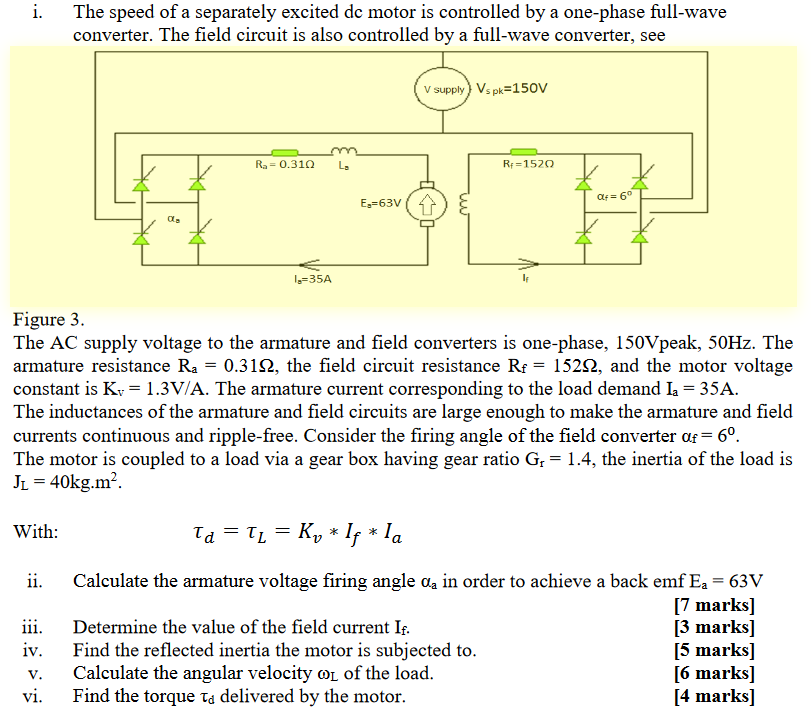i. The speed of a separately excited dc motor is controlled by a one-phase full-wave converter. The field circuit is also controlled by a full-wave converter, see V supply) Vs pk-150V Ra-0.310 L Rf 152O E-63V α. 1-35A Figure 3 The AC supply voltage to the armature and field converters is one-phase, 150Vpeak, 50Hz. The armature resistance Ra-0.31Ω, the field circuit resistance Rf-152Ω, and the motor voltage constant is Kv-1.3V/A. The armature current corresponding to the load demand la-35A The inductances of the armature and field circuits are large enough to make the armature and field currents continuous and ripple-free. Consider the firing angle of the field converter f-60. The motor is coupled to a load via a gear box having gear ratio Gr 1.4, the inertia of the load is Ji- 40kg.m2 With ii. Calculate the armature voltage firing angle aa in order to achieve a back emf Ea- 63V [7 marks] [3 marks] [5 marks] [6 marks] [4 marks] 111, Determine the value of the field current If iv. Find the reflected inertia the motor is subjected to V. Calculate the angular velocity ωL of the load vi. Find the torque td delivered by the motor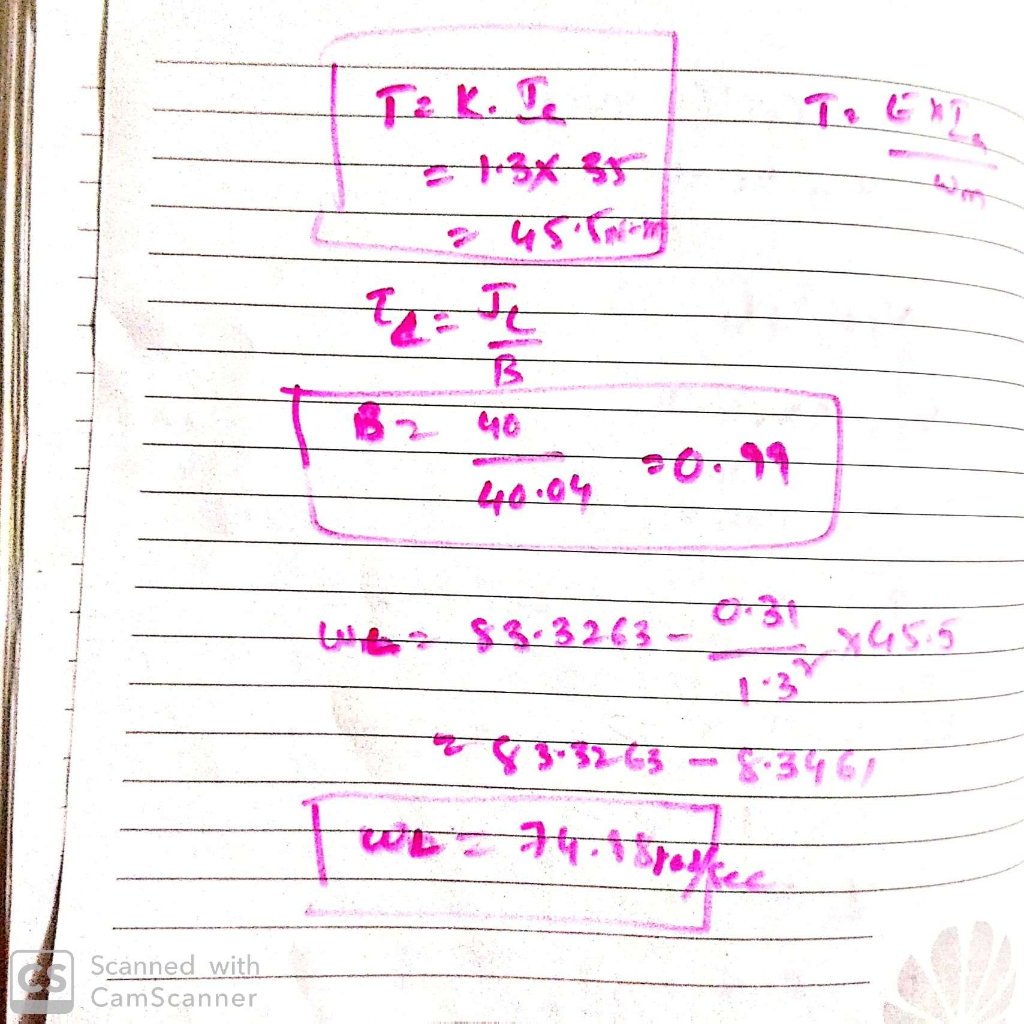##### Add Answer of: i. The speed of a separately excited dc motor is controlled by a one-phase full-wave converter. The field circuit is also controlled by a full-wave converter, see V supply) Vs pk-150V Ra-0.310 L Rf 1...
Similar Homework Help Questions
• ### The speed of a separately excited de motor is controlled by a one-phase full-wave converter. The field circuit is also controlled by a full-wave converter and the field current is set to its maximum...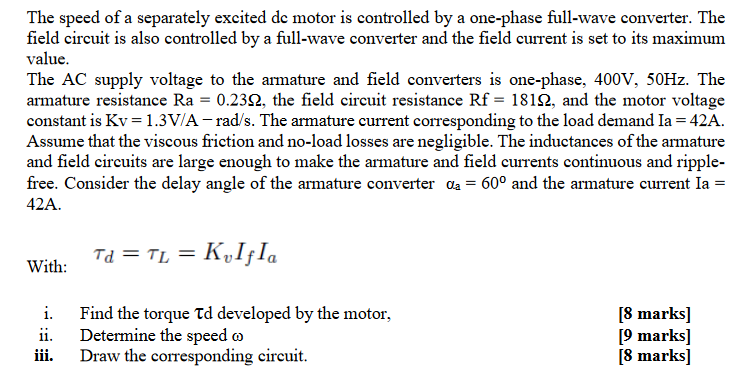The speed of a separately excited de motor is controlled by a one-phase full-wave converter. The field circuit is also controlled by a full-wave converter and the field current is set to its maximum value. The AC supply voltage to the armature and field converters is one-phase, 400V, 50Hz. The armature resistance Ra 0.232, the field circuit resistance Rf- 1812, and the motor voltage constant is Kv 1.3V/A - rad/s. The armature current corresponding to the load demand Ia 42A...

• ### A separately excited dc motor is fed by a 3-phase, 6-pulse converter shown in figure Q5. The 3-phase line is a 500 V, 6...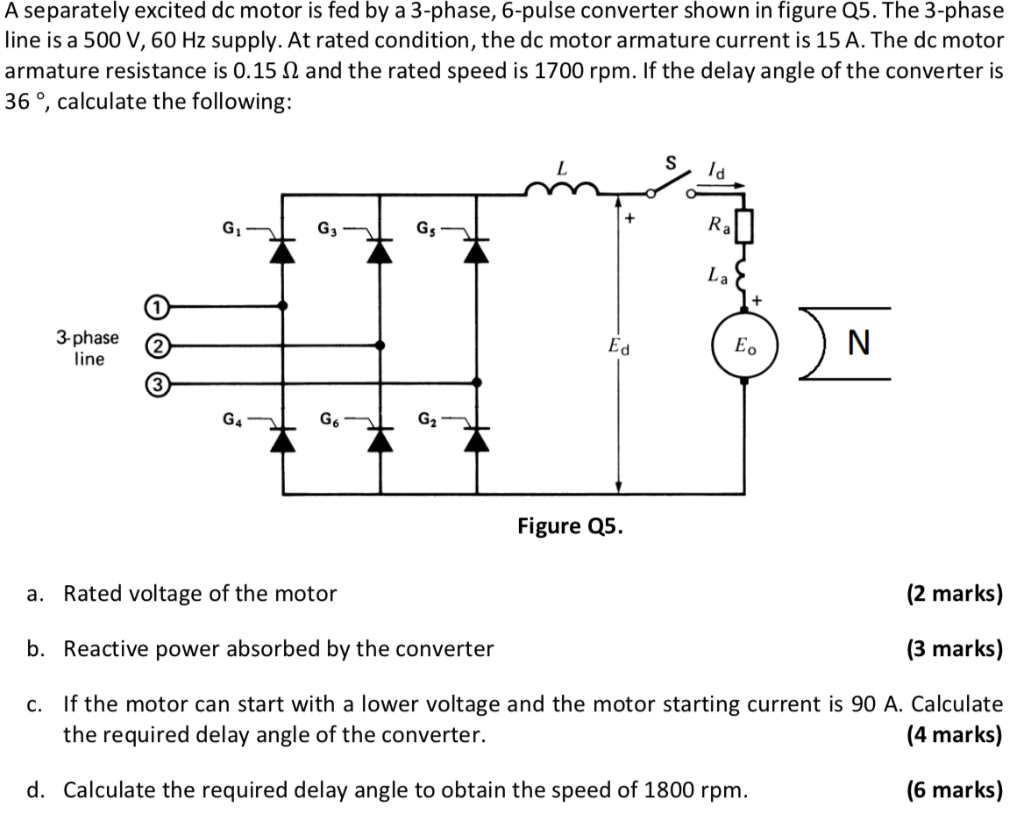A separately excited dc motor is fed by a 3-phase, 6-pulse converter shown in figure Q5. The 3-phase line is a 500 V, 60 Hz supply. At rated condition, the dc motor armature current is 15 A. The dc motor armature resistance is 0.15 Ω and the rated speed is 1700 rpm. If the delay angle of the converter is 36 °, calculate the following: 3-phase (2 0 line Figure Q5. (2 marks) Rated voltage of the motor a. b....

• ### 3. A controlled single-phase fiull-wave controlled rectifier is connected in a de motor drive in Figure...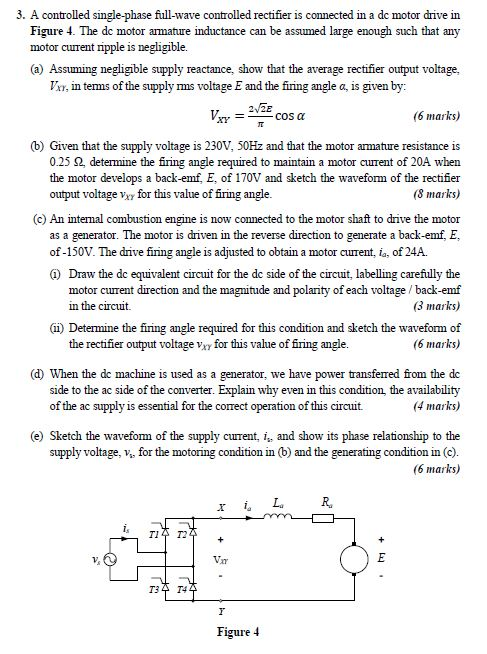3. A controlled single-phase fiull-wave controlled rectifier is connected in a de motor drive in Figure 4. The de motor armature inductance can be assumed large enough such that any motor curent ripple is negligible. (a) Assuming negligible supply reactance, show that the average rectifier output voltage V, in tems of the supply ms voltage E and the firing angle a, is given by 2/2E (6 marks) cos a _ (b) Given that the supply voltage is 230V, 50HZ and...

• ### please solve this problem with detail explainations. Thanks Problem # 1 If the available power source is a three-phase, 60 Hz, ac supply and a separately excited DC motor is to be fed from this sourc...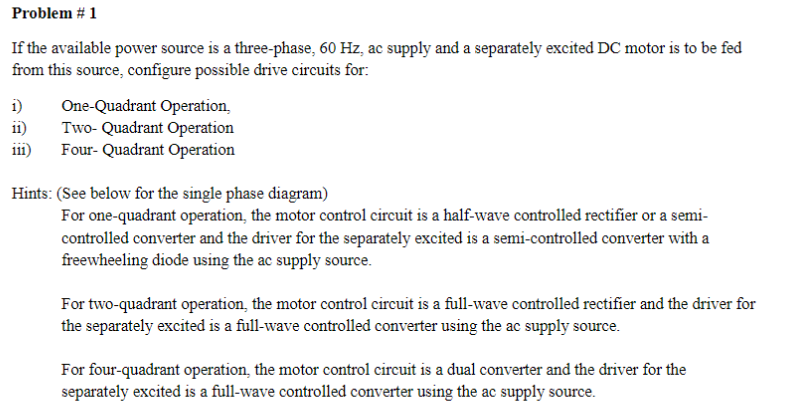please solve this problem with detail explainations. Thanks Problem # 1 If the available power source is a three-phase, 60 Hz, ac supply and a separately excited DC motor is to be fed from this source, configure possible drive circuits for iOne-Quadrant Operation, i) Two-Quadrant Operation ) Four- Quadrant Operation Hints: (See below for the single phase diagram) For one-quadrant operation, the motor control circuit is a half-wave controlled rectifier or a se controlled converter and the driver for the...

• ### Problem 2 A separately excited DC motor is characterized by the followng intormation Armature res...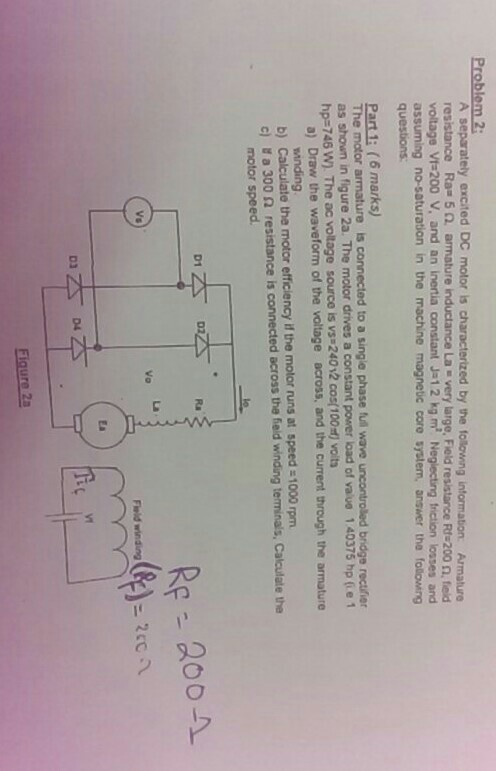Problem 2 A separately excited DC motor is characterized by the followng intormation Armature resistance Ra-5 2 armature inductance La . very large, Field resistance RS200 Ω, ted voltage Vt 200 V, and an inertia constant J-12 kg m Neglecting friction losses and assuming no-saturation in the machine magnetic core system, answer the folowing questions: Part 1: (6 marks) he motor a as shown in figure 2a. The motor drives a constant power load of vaue 1 40375 hp (ie...

• ### Open Book Exam A 220 V, 1000 V dc-sourc rpm, separately dc motor is fed from a dc-dc converter th...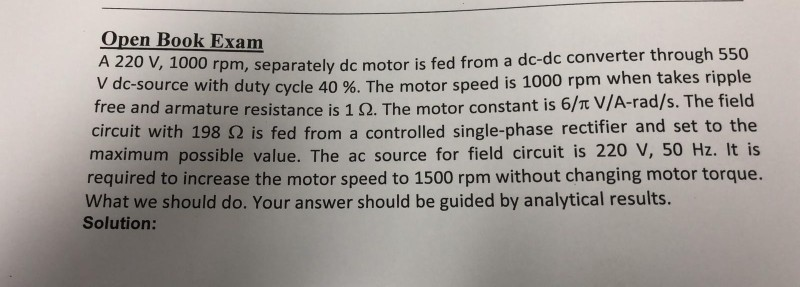Open Book Exam A 220 V, 1000 V dc-sourc rpm, separately dc motor is fed from a dc-dc converter through 550 e with duty cycle 40 %. The motor speed is 1000 rpm when takes ripple free and armature resistance is 1 Ω. The motor constant is 6/π V/A-rad/s. The field circuit with 198 Ω is fed from a controlled single-phase rectifier and set to the maximum possible value. The ac source for field circuit is 220 V, 50 Hz....

• ### Shown in Figure 1(a) is a separately excited generator. It is known that Rf-250, Ra- 10 Ω, v,-100...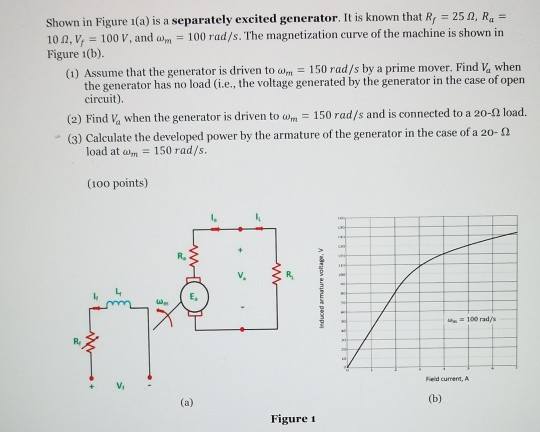shown in Figure 1(a) is a separately excited generator. It is known that Rf-250, Ra- 10 Ω, v,-100 V, and ahn-100 rad/s. The magnetization curve of the machine is shown in Figure 1(b). when (1) Assume that the generator is driven to Wm-150 rad/s by a prime mover. Find the generator has no load (i.e., the voltage generated by the generator in the case of open circuit) (2) Find Va when the generator is driven to am-150 rad/s and is...

• ### A three phase induction motor draws a starting current which is five times the full load...

A three phase induction motor draws a starting current which is five times the full load current with direct on line starting, while developing twice the full load torque. Q2.1. Based on the two facts that starting current is proportional to the applied voltage and that the starting torque is proportional to the square of the applied voltage, determine voltage (as a per cent of rated voltage) required to obtain a starting torque equalling the full load torque. (10 marks)...

• ### 2.3 (20 points) A separately-excited de motor is mechanically coupled to a three-phase, 4-pole, 30-kVA, 460-V, round-rotor synchronous generator. The de motor is connected to a constant 230-V de...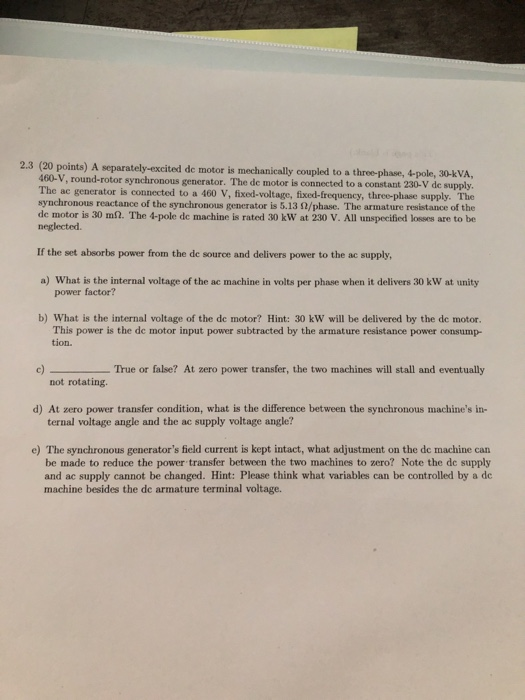2.3 (20 points) A separately-excited de motor is mechanically coupled to a three-phase, 4-pole, 30-kVA, 460-V, round-rotor synchronous generator. The de motor is connected to a constant 230-V de supply The ac generator is connected to a 460 V, fixed-voltage, fixed-frequency, three-phase supply. The synchronous reactance of the synchronous generator is 513 Ω/phase. The armature resistance of the de motor is 30 m2. The 4-pole de machine is rated 30 kW at 230 V. All unspecified losses are to be...

• ### Please dont copy paste from other user Question. Need to learn step by step solution. 5. A three-phase half-wave converter is operated from a three-phase 400 V and 50 Hz supply and the load resistanc...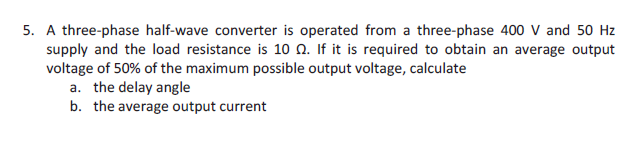Please dont copy paste from other user Question. Need to learn step by step solution. 5. A three-phase half-wave converter is operated from a three-phase 400 V and 50 Hz supply and the load resistance is 10 Q. If it is required to obtain an average output voltage of 50% of the maximum possible output voltage, calculate the delay angle the average output current a. b. 5. A three-phase half-wave converter is operated from a three-phase 400 V and 50...

Need Online Homework Help?